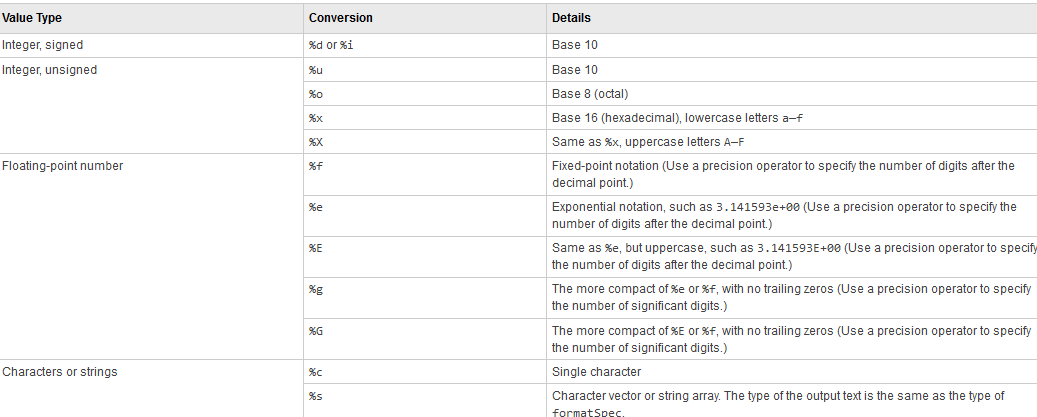Showing posts with label fprint function in MATLAB. Show all posts
Showing posts with label fprint function in MATLAB. Show all posts

## fprintf command And fprintf format in MATLAB

•  The fprintf command in MATLAB Programming use to displays formatted text which is centered on the icon and this fprint function can display formatSpec with a contents of var.
• `formatSpec`  in MATLAB can be a character vector with the single quotes, or a string scalar.

## Formatting for the fprint function in MATLAB : -

•  formatting is starts with the a percentage sign, % and it will end with the conversion character.
• Remember conversion character is required in formatting Operator.
• But you can use the  flags, field , identifier,width, and subtype,precision operators between % and conversion character.

## List for the Conversion CharacterConversion Character in MATLAB

## Example of fprint function in MATLAB

```The command

fprintf('YES YES');

displays this text 'YES YES' on the icon.

fprintf('YES YES = %d',16);

uses the decimal notation format (%d) to display the variable 16.```

## Using fprintf in MATLAB With Function

Let's see this example for define a function in MATLAB : -
```function []= fun2let(n)
if n > 90.00